# 3.1 Vector-valued functions and space curves  (Page 4/12)

 Page 4 / 12

Calculate $\underset{t\to -2}{\text{lim}}\phantom{\rule{0.1em}{0ex}}\text{r}\left(t\right)$ for the function $\text{r}\left(t\right)=\sqrt{{t}^{2}-3t-1}\phantom{\rule{0.1em}{0ex}}\text{i}+\left(4t+3\right)\phantom{\rule{0.1em}{0ex}}\text{j}+\text{sin}\phantom{\rule{0.2em}{0ex}}\frac{\left(t+1\right)\pi }{2}\phantom{\rule{0.1em}{0ex}}\text{k}.$

$\underset{t\to -2}{\text{lim}}\phantom{\rule{0.1em}{0ex}}\text{r}\left(t\right)=3\phantom{\rule{0.1em}{0ex}}\text{i}-5\phantom{\rule{0.1em}{0ex}}\text{j}-\text{k}$

Now that we know how to calculate the limit of a vector-valued function, we can define continuity at a point for such a function.

## Definition

Let f, g, and h be functions of t. Then, the vector-valued function $\text{r}\left(t\right)=f\left(t\right)\phantom{\rule{0.1em}{0ex}}\text{i}+g\left(t\right)\phantom{\rule{0.1em}{0ex}}\text{j}$ is continuous at point $t=a$ if the following three conditions hold:

1. $\text{r}\left(a\right)$ exists
2. $\underset{t\to a}{\text{lim}}\phantom{\rule{0.1em}{0ex}}\text{r}\left(t\right)$ exists
3. $\underset{t\to a}{\text{lim}}\phantom{\rule{0.1em}{0ex}}\text{r}\left(t\right)=\text{r}\left(a\right)$

Similarly, the vector-valued function $\text{r}\left(t\right)=f\left(t\right)\phantom{\rule{0.1em}{0ex}}\text{i}+g\left(t\right)\phantom{\rule{0.1em}{0ex}}\text{j}+h\left(t\right)\phantom{\rule{0.1em}{0ex}}\text{k}$ is continuous at point $t=a$ if the following three conditions hold:

1. $\text{r}\left(a\right)$ exists
2. $\underset{t\to a}{\text{lim}}\phantom{\rule{0.1em}{0ex}}\text{r}\left(t\right)$ exists
3. $\underset{t\to a}{\text{lim}}\phantom{\rule{0.1em}{0ex}}\text{r}\left(t\right)=\text{r}\left(a\right)$

## Key concepts

• A vector-valued function is a function of the form $\text{r}\left(t\right)=f\left(t\right)\phantom{\rule{0.1em}{0ex}}\text{i}+g\left(t\right)\phantom{\rule{0.1em}{0ex}}\text{j}$ or $\text{r}\left(t\right)=f\left(t\right)\phantom{\rule{0.1em}{0ex}}\text{i}+g\left(t\right)\phantom{\rule{0.1em}{0ex}}\text{j}+h\left(t\right)\phantom{\rule{0.1em}{0ex}}\text{k},$ where the component functions f, g, and h are real-valued functions of the parameter t .
• The graph of a vector-valued function of the form $\text{r}\left(t\right)=f\left(t\right)\phantom{\rule{0.1em}{0ex}}\text{i}+g\left(t\right)\phantom{\rule{0.1em}{0ex}}\text{j}$ is called a plane curve . The graph of a vector-valued function of the form $\text{r}\left(t\right)=f\left(t\right)\phantom{\rule{0.1em}{0ex}}\text{i}+g\left(t\right)\phantom{\rule{0.1em}{0ex}}\text{j}+h\left(t\right)\phantom{\rule{0.1em}{0ex}}\text{k}$ is called a space curve .
• It is possible to represent an arbitrary plane curve by a vector-valued function.
• To calculate the limit of a vector-valued function, calculate the limits of the component functions separately.

## Key equations

• Vector-valued function
$\text{r}\left(t\right)=f\left(t\right)\phantom{\rule{0.1em}{0ex}}\text{i}+g\left(t\right)\phantom{\rule{0.1em}{0ex}}\text{j}\phantom{\rule{0.5em}{0ex}}\text{or}\phantom{\rule{0.5em}{0ex}}\text{r}\left(t\right)=f\left(t\right)\phantom{\rule{0.1em}{0ex}}\text{i}+g\left(t\right)\phantom{\rule{0.1em}{0ex}}\text{j}+h\left(t\right)\phantom{\rule{0.1em}{0ex}}\text{k},\phantom{\rule{0.2em}{0ex}}\text{or}\phantom{\rule{0.5em}{0ex}}\text{r}\left(t\right)=⟨f\left(t\right),g\left(t\right)⟩\phantom{\rule{0.2em}{0ex}}\text{or}\phantom{\rule{0.5em}{0ex}}\text{r}\left(t\right)=⟨f\left(t\right),g\left(t\right),h\left(t\right)⟩$
• Limit of a vector-valued function
$\underset{t\to a}{\text{lim}}\phantom{\rule{0.1em}{0ex}}\text{r}\left(t\right)=\left[\underset{t\to a}{\text{lim}}f\left(t\right)\right]\phantom{\rule{0.1em}{0ex}}\text{i}+\left[\underset{t\to a}{\text{lim}}g\left(t\right)\right]\phantom{\rule{0.1em}{0ex}}\text{j}\phantom{\rule{0.2em}{0ex}}\text{or}\phantom{\rule{0.2em}{0ex}}\underset{t\to a}{\text{lim}}\phantom{\rule{0.1em}{0ex}}\text{r}\left(t\right)=\left[\underset{t\to a}{\text{lim}}f\left(t\right)\right]\phantom{\rule{0.1em}{0ex}}\text{i}+\left[\underset{t\to a}{\text{lim}}g\left(t\right)\right]\phantom{\rule{0.1em}{0ex}}\text{j}+\left[\underset{t\to a}{\text{lim}}h\left(t\right)\right]\phantom{\rule{0.1em}{0ex}}\text{k}$

Give the component functions $x=f\left(t\right)$ and $y=g\left(t\right)$ for the vector-valued function $\text{r}\left(t\right)=3\phantom{\rule{0.1em}{0ex}}\text{sec}\phantom{\rule{0.1em}{0ex}}t\phantom{\rule{0.1em}{0ex}}\text{i}+2\phantom{\rule{0.1em}{0ex}}\text{tan}\phantom{\rule{0.1em}{0ex}}t\phantom{\rule{0.1em}{0ex}}\text{j}.$

$f\left(t\right)=3\phantom{\rule{0.1em}{0ex}}\text{sec}\phantom{\rule{0.1em}{0ex}}t,g\left(t\right)=2\phantom{\rule{0.1em}{0ex}}\text{tan}\phantom{\rule{0.1em}{0ex}}t$

Given $\text{r}\left(t\right)=3\phantom{\rule{0.1em}{0ex}}\text{sec}\phantom{\rule{0.1em}{0ex}}t\phantom{\rule{0.1em}{0ex}}\text{i}+2\phantom{\rule{0.1em}{0ex}}\text{tan}\phantom{\rule{0.1em}{0ex}}t\phantom{\rule{0.1em}{0ex}}\text{j},$ find the following values (if possible).

1. $\text{r}\left(\frac{\pi }{4}\right)$
2. $\text{r}\left(\pi \right)$
3. $\text{r}\left(\frac{\pi }{2}\right)$

Sketch the curve of the vector-valued function $\text{r}\left(t\right)=3\phantom{\rule{0.1em}{0ex}}\text{sec}\phantom{\rule{0.1em}{0ex}}t\phantom{\rule{0.1em}{0ex}}\text{i}+2\phantom{\rule{0.1em}{0ex}}\text{tan}\phantom{\rule{0.1em}{0ex}}t\phantom{\rule{0.1em}{0ex}}\text{j}$ and give the orientation of the curve. Sketch asymptotes as a guide to the graph.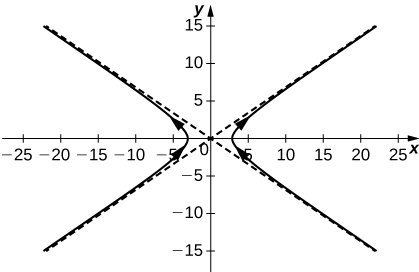Evaluate $\underset{t\to 0}{\text{lim}}⟨{e}^{t}\phantom{\rule{0.1em}{0ex}}\text{i}+\frac{\text{sin}\phantom{\rule{0.1em}{0ex}}t}{t}\phantom{\rule{0.1em}{0ex}}\text{j}+{e}^{\text{−}t}\phantom{\rule{0.1em}{0ex}}\text{k}⟩.$

Given the vector-valued function $\text{r}\left(t\right)=⟨\text{cos}\phantom{\rule{0.1em}{0ex}}t,\text{sin}\phantom{\rule{0.1em}{0ex}}t⟩,$ find the following values:

1. $\underset{t\to \frac{\pi }{4}}{\text{lim}}\phantom{\rule{0.1em}{0ex}}\text{r}\left(t\right)$
2. $\text{r}\left(\frac{\pi }{3}\right)$
3. Is $\text{r}\left(t\right)$ continuous at $t=\frac{\pi }{3}?$
4. Graph $\text{r}\left(t\right).$

a. $⟨\frac{\sqrt{2}}{2},\frac{\sqrt{2}}{2}⟩,$ b. $⟨\frac{1}{2},\frac{\sqrt{3}}{2}⟩,$ c. Yes, the limit as t approaches $\pi \text{/}3$ is equal to $\text{r}\left(\pi \text{/}3\right),$ d.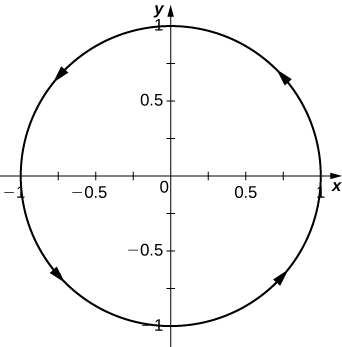Given the vector-valued function $\text{r}\left(t\right)=⟨t,{t}^{2}+1⟩,$ find the following values:

1. $\underset{t\to -3}{\text{lim}}\phantom{\rule{0.1em}{0ex}}\text{r}\left(t\right)$
2. $\text{r}\left(-3\right)$
3. Is $\text{r}\left(t\right)$ continuous at $x=-3?$
4. $\text{r}\left(t+2\right)-\text{r}\left(t\right)$

Let $\text{r}\left(t\right)={e}^{t}\phantom{\rule{0.1em}{0ex}}\text{i}+\text{sin}\phantom{\rule{0.1em}{0ex}}t\phantom{\rule{0.1em}{0ex}}\text{j}+\text{ln}\phantom{\rule{0.1em}{0ex}}t\phantom{\rule{0.1em}{0ex}}\text{k}.$ Find the following values:

1. $\text{r}\left(\frac{\pi }{4}\right)$
2. $\underset{t\to \pi \text{/}4}{\text{lim}}\phantom{\rule{0.1em}{0ex}}\text{r}\left(t\right)$
3. Is $\text{r}\left(t\right)$ continuous at $t=t=\frac{\pi }{4}?$

a. $⟨{e}^{\pi \text{/}4},\frac{\sqrt{2}}{2},\text{ln}\left(\frac{\pi }{4}\right)⟩;$ b. $⟨{e}^{\pi \text{/}4},\frac{\sqrt{2}}{2},\text{ln}\left(\frac{\pi }{4}\right)⟩;$ c. Yes

Find the limit of the following vector-valued functions at the indicated value of t .

$\underset{t\to 4}{\text{lim}}⟨\sqrt{t-3},\frac{\sqrt{t}-2}{t-4},\text{tan}\left(\frac{\pi }{t}\right)⟩$

$\underset{t\to \pi \text{/}2}{\text{lim}}\phantom{\rule{0.1em}{0ex}}\text{r}\left(t\right)$ for $\text{r}\left(t\right)={e}^{t}\phantom{\rule{0.1em}{0ex}}\text{i}+\text{sin}\phantom{\rule{0.1em}{0ex}}t\phantom{\rule{0.1em}{0ex}}\text{j}+\text{ln}\phantom{\rule{0.1em}{0ex}}t\phantom{\rule{0.1em}{0ex}}\text{k}$

$⟨{e}^{\pi \text{/}2},1,\text{ln}\left(\frac{\pi }{2}\right)⟩$

$\underset{t\to \infty }{\text{lim}}⟨{e}^{-2t},\frac{2t+3}{3t-1},\text{arctan}\left(2t\right)⟩$

$\underset{t\to {e}^{2}}{\text{lim}}⟨t\phantom{\rule{0.1em}{0ex}}\text{ln}\left(t\right),\frac{\text{ln}\phantom{\rule{0.1em}{0ex}}t}{{t}^{2}},\sqrt{\text{ln}\phantom{\rule{0.1em}{0ex}}\left({t}^{2}\right)}⟩$

$2{e}^{2}\phantom{\rule{0.1em}{0ex}}\text{i}+\frac{2}{{e}^{4}}\phantom{\rule{0.1em}{0ex}}\text{j}+2\phantom{\rule{0.1em}{0ex}}\text{k}$

$\underset{t\to \pi \text{/}6}{\text{lim}}⟨{\text{cos}}^{2}t,{\text{sin}}^{2}t,1⟩$

$\underset{t\to \infty }{\text{lim}}\phantom{\rule{0.1em}{0ex}}\text{r}\left(t\right)$ for $\text{r}\left(t\right)=2{e}^{\text{−}t}\phantom{\rule{0.1em}{0ex}}\text{i}+{e}^{\text{−}t}\phantom{\rule{0.1em}{0ex}}\text{j}+\text{ln}\left(t-1\right)\phantom{\rule{0.1em}{0ex}}\text{k}$

The limit does not exist because the limit of $\text{ln}\left(t-1\right)$ as t approaches infinity does not exist.

Describe the curve defined by the vector-valued function $\text{r}\left(t\right)=\left(1+t\right)\phantom{\rule{0.1em}{0ex}}\text{i}+\left(2+5t\right)\phantom{\rule{0.1em}{0ex}}\text{j}+\left(-1+6t\right)\phantom{\rule{0.1em}{0ex}}\text{k}.$

Find the domain of the vector-valued functions.

Domain: $\text{r}\left(t\right)=⟨{t}^{2},\text{tan}\phantom{\rule{0.1em}{0ex}}t,\text{ln}\phantom{\rule{0.1em}{0ex}}t⟩$

$t>0,t\ne \left(2k+1\right)\frac{\pi }{2},$ where k is an integer

Domain: $\text{r}\left(t\right)=⟨{t}^{2},\sqrt{t-3},\frac{3}{2t+1}⟩$

Domain: $\text{r}\left(t\right)=⟨\text{csc}\left(t\right),\frac{1}{\sqrt{t-3}},\text{ln}\left(t-2\right)⟩$

$t>3,t\ne n\pi ,$ where n is an integer

Let $\text{r}\left(t\right)=⟨\text{cos}\phantom{\rule{0.1em}{0ex}}t,t,\text{sin}\phantom{\rule{0.1em}{0ex}}t⟩$ and use it to answer the following questions.

For what values of t is $\text{r}\left(t\right)$ continuous?

Sketch the graph of $\text{r}\left(t\right).$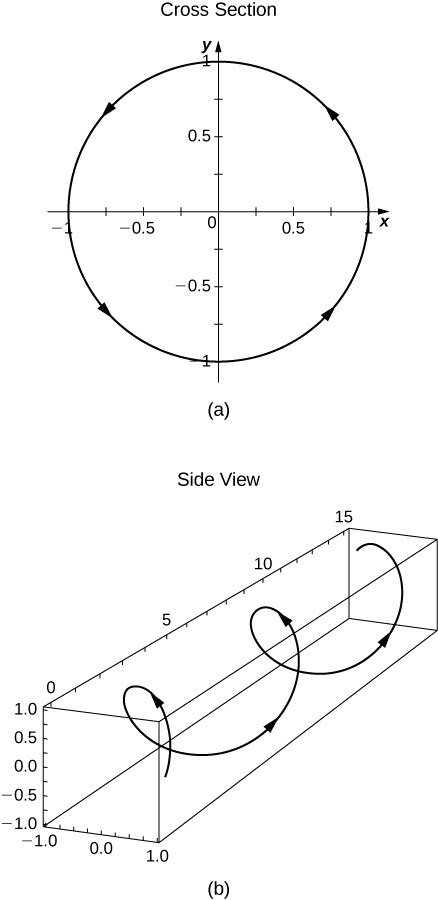Find the domain of $\text{r}\left(t\right)=2{e}^{\text{−}t}\phantom{\rule{0.1em}{0ex}}\text{i}+{e}^{\text{−}t}\phantom{\rule{0.1em}{0ex}}\text{j}+\text{ln}\left(t-1\right)\phantom{\rule{0.1em}{0ex}}\text{k}.$

For what values of t is $\text{r}\left(t\right)=2{e}^{\text{−}t}\phantom{\rule{0.1em}{0ex}}\text{i}+{e}^{\text{−}t}\phantom{\rule{0.1em}{0ex}}\text{j}+\text{ln}\left(t-1\right)\phantom{\rule{0.1em}{0ex}}\text{k}$ continuous?

All t such that $t\in \left(1,\infty \right)$

Eliminate the parameter t , write the equation in Cartesian coordinates, then sketch the graphs of the vector-valued functions. ( Hint: Let $x=2t$ and $y={t}^{2}.$ Solve the first equation for x in terms of t and substitute this result into the second equation.)

$\text{r}\left(t\right)=2t\phantom{\rule{0.1em}{0ex}}\text{i}+{t}^{2}\phantom{\rule{0.1em}{0ex}}\text{j}$

$\text{r}\left(t\right)={t}^{3}\phantom{\rule{0.1em}{0ex}}\text{i}+2t\phantom{\rule{0.1em}{0ex}}\text{j}$

$y=2\sqrt{x},$ a variation of the cube-root function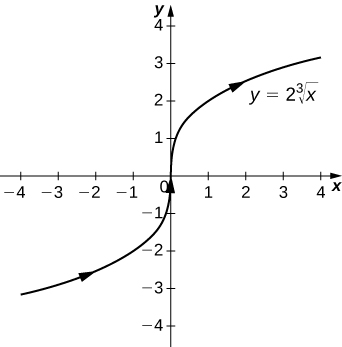$\text{r}\left(t\right)=2\left(\text{sinh}\phantom{\rule{0.1em}{0ex}}t\right)\phantom{\rule{0.1em}{0ex}}\text{i}+2\left(\text{cosh}\phantom{\rule{0.1em}{0ex}}t\right)\phantom{\rule{0.1em}{0ex}}\text{j},t>0$

$\text{r}\left(t\right)=3\left(\text{cos}\phantom{\rule{0.1em}{0ex}}t\right)\phantom{\rule{0.1em}{0ex}}\text{i}+3\left(\text{sin}\phantom{\rule{0.1em}{0ex}}t\right)\phantom{\rule{0.1em}{0ex}}\text{j}$

${x}^{2}+{y}^{2}=9,$ a circle centered at $\left(0,0\right)$ with radius 3, and a counterclockwise orientation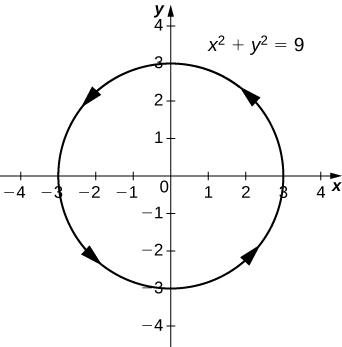$\text{r}\left(t\right)=⟨3\phantom{\rule{0.1em}{0ex}}\text{sin}\phantom{\rule{0.1em}{0ex}}t,3\phantom{\rule{0.1em}{0ex}}\text{cos}\phantom{\rule{0.1em}{0ex}}t⟩$

Use a graphing utility to sketch each of the following vector-valued functions:

[T] $\text{r}\left(t\right)=2\phantom{\rule{0.1em}{0ex}}\text{cos}\phantom{\rule{0.1em}{0ex}}{t}^{2}\phantom{\rule{0.1em}{0ex}}\text{i}+\left(2-\sqrt{t}\right)\phantom{\rule{0.1em}{0ex}}\text{j}$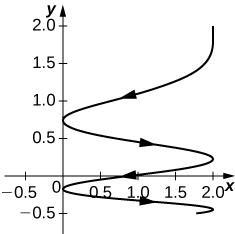[T] $\text{r}\left(t\right)=⟨{e}^{\text{cos}\left(3t\right)},{e}^{\text{−}\text{sin}\left(t\right)}⟩$

[T] $\text{r}\left(t\right)=⟨2-\text{sin}\left(2t\right),3+2\phantom{\rule{0.1em}{0ex}}\text{cos}\phantom{\rule{0.1em}{0ex}}t⟩$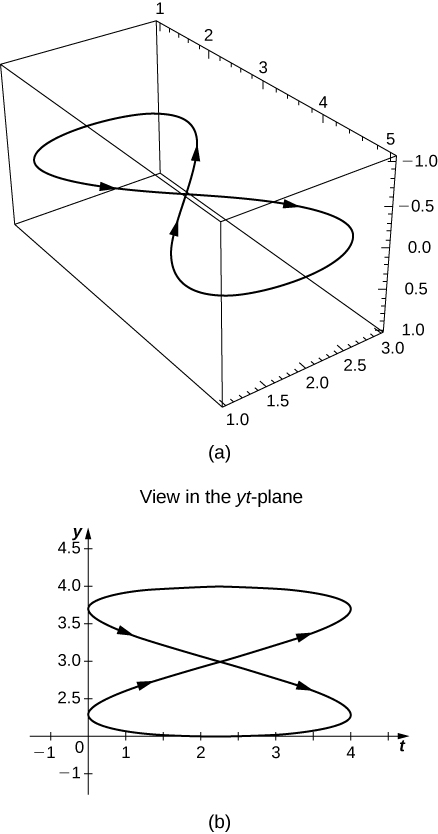Find a vector-valued function that traces out the given curve in the indicated direction.

$4{x}^{2}+9{y}^{2}=36;$ clockwise and counterclockwise

$\text{r}\left(t\right)=⟨t,{t}^{2}⟩;$ from left to right

For left to right, $y={x}^{2},$ where t increases

The line through P and Q where P is $\left(1,4,-2\right)$ and Q is $\left(3,9,6\right)$

Consider the curve described by the vector-valued function $\text{r}\left(t\right)=\left(50{e}^{\text{−}t}\text{cos}\phantom{\rule{0.1em}{0ex}}t\right)\phantom{\rule{0.1em}{0ex}}\text{i}+\left(50{e}^{\text{−}t}\text{sin}\phantom{\rule{0.1em}{0ex}}t\right)\phantom{\rule{0.1em}{0ex}}\text{j}+\left(5-5{e}^{\text{−}t}\right)\phantom{\rule{0.1em}{0ex}}\text{k}.$

What is the initial point of the path corresponding to $\text{r}\left(0\right)?$

$\left(50,0,0\right)$

What is $\underset{t\to \infty }{\text{lim}}\phantom{\rule{0.1em}{0ex}}\text{r}\left(t\right)?$

[T] Use technology to sketch the curve.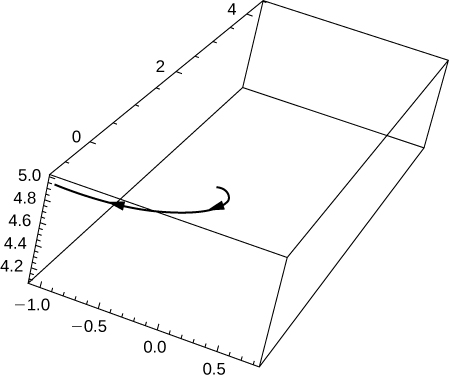Eliminate the parameter t to show that $z=5-\frac{r}{10}$ where $r={x}^{2}+{y}^{2}.$

[T] Let $r\left(t\right)=\text{cos}\phantom{\rule{0.1em}{0ex}}t\phantom{\rule{0.1em}{0ex}}\text{i}+\text{sin}\phantom{\rule{0.1em}{0ex}}t\phantom{\rule{0.1em}{0ex}}\text{j}+0.3\phantom{\rule{0.1em}{0ex}}\text{sin}\left(2t\right)\phantom{\rule{0.1em}{0ex}}\text{k}.$ Use technology to graph the curve (called the roller-coaster curve ) over the interval $\left[0,2\pi \right).$ Choose at least two views to determine the peaks and valleys.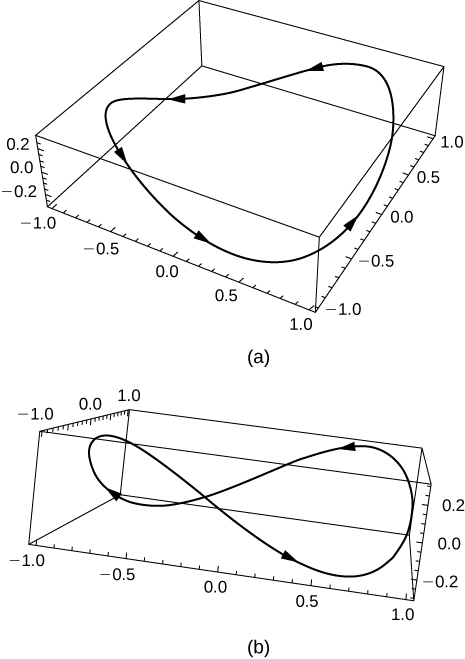[T] Use the result of the preceding problem to construct an equation of a roller coaster with a steep drop from the peak and steep incline from the “valley.” Then, use technology to graph the equation.

Use the results of the preceding two problems to construct an equation of a path of a roller coaster with more than two turning points (peaks and valleys).

One possibility is $r\left(t\right)=\text{cos}\phantom{\rule{0.1em}{0ex}}t\phantom{\rule{0.1em}{0ex}}\text{i}+\text{sin}\phantom{\rule{0.1em}{0ex}}t\phantom{\rule{0.1em}{0ex}}\text{j}+\text{sin}\left(4t\right)\phantom{\rule{0.1em}{0ex}}\text{k}.$ By increasing the coefficient of t in the third component, the number of turning points will increase.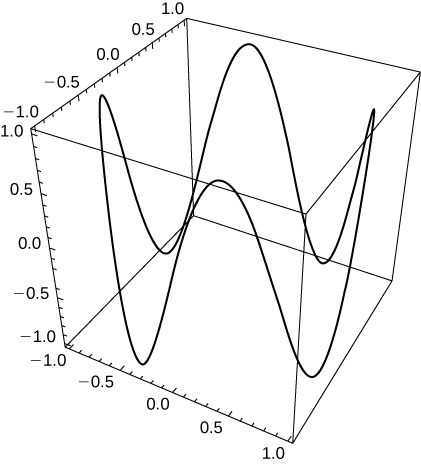1. Graph the curve $\text{r}\left(t\right)=\left(4+\text{cos}\left(18t\right)\right)\text{cos}\left(t\right)\phantom{\rule{0.1em}{0ex}}\text{i}+\left(4+\text{cos}\left(18t\right)\text{sin}\left(t\right)\right)\phantom{\rule{0.1em}{0ex}}\text{j}+0.3\phantom{\rule{0.1em}{0ex}}\text{sin}\left(18t\right)\phantom{\rule{0.1em}{0ex}}\text{k}$ using two viewing angles of your choice to see the overall shape of the curve.
2. Does the curve resemble a “slinky”?
3. What changes to the equation should be made to increase the number of coils of the slinky?

#### Questions & Answers

how can chip be made from sand
Eke Reply
is this allso about nanoscale material
Almas
are nano particles real
Missy Reply
yeah
Joseph
Hello, if I study Physics teacher in bachelor, can I study Nanotechnology in master?
Lale Reply
no can't
Lohitha
where is the latest information on a no technology how can I find it
William
currently
William
where we get a research paper on Nano chemistry....?
Maira Reply
nanopartical of organic/inorganic / physical chemistry , pdf / thesis / review
Ali
what are the products of Nano chemistry?
Maira Reply
There are lots of products of nano chemistry... Like nano coatings.....carbon fiber.. And lots of others..
learn
Even nanotechnology is pretty much all about chemistry... Its the chemistry on quantum or atomic level
learn
Google
da
no nanotechnology is also a part of physics and maths it requires angle formulas and some pressure regarding concepts
Bhagvanji
hey
Giriraj
Preparation and Applications of Nanomaterial for Drug Delivery
Hafiz Reply
revolt
da
Application of nanotechnology in medicine
has a lot of application modern world
Kamaluddeen
yes
narayan
what is variations in raman spectra for nanomaterials
Jyoti Reply
ya I also want to know the raman spectra
Bhagvanji
I only see partial conversation and what's the question here!
Crow Reply
what about nanotechnology for water purification
RAW Reply
please someone correct me if I'm wrong but I think one can use nanoparticles, specially silver nanoparticles for water treatment.
Damian
yes that's correct
Professor
I think
Professor
Nasa has use it in the 60's, copper as water purification in the moon travel.
Alexandre
nanocopper obvius
Alexandre
what is the stm
Brian Reply
is there industrial application of fullrenes. What is the method to prepare fullrene on large scale.?
Rafiq
industrial application...? mmm I think on the medical side as drug carrier, but you should go deeper on your research, I may be wrong
Damian
How we are making nano material?
LITNING Reply
what is a peer
LITNING Reply
What is meant by 'nano scale'?
LITNING Reply
What is STMs full form?
LITNING
scanning tunneling microscope
Sahil
how nano science is used for hydrophobicity
Santosh
Do u think that Graphene and Fullrene fiber can be used to make Air Plane body structure the lightest and strongest. Rafiq
Rafiq
what is differents between GO and RGO?
Mahi
what is simplest way to understand the applications of nano robots used to detect the cancer affected cell of human body.? How this robot is carried to required site of body cell.? what will be the carrier material and how can be detected that correct delivery of drug is done Rafiq
Rafiq
if virus is killing to make ARTIFICIAL DNA OF GRAPHENE FOR KILLED THE VIRUS .THIS IS OUR ASSUMPTION
Anam
analytical skills graphene is prepared to kill any type viruses .
Anam
Any one who tell me about Preparation and application of Nanomaterial for drug Delivery
Hafiz
what is Nano technology ?
Bob Reply
write examples of Nano molecule?
Bob
The nanotechnology is as new science, to scale nanometric
brayan
nanotechnology is the study, desing, synthesis, manipulation and application of materials and functional systems through control of matter at nanoscale
Damian
how did you get the value of 2000N.What calculations are needed to arrive at it
Smarajit Reply
Privacy Information Security Software Version 1.1a
Good
can you provide the details of the parametric equations for the lines that defince doubly-ruled surfeces (huperbolids of one sheet and hyperbolic paraboloid). Can you explain each of the variables in the equations?
Radek Reply

### Read also:

#### Get Jobilize Job Search Mobile App in your pocket Now!

Source:  OpenStax, Calculus volume 3. OpenStax CNX. Feb 05, 2016 Download for free at http://legacy.cnx.org/content/col11966/1.2
Google Play and the Google Play logo are trademarks of Google Inc.

Notification Switch

Would you like to follow the 'Calculus volume 3' conversation and receive update notifications?By Stephen VoronBy Ryan LoweBy Jonathan LongBy OpenStaxBy David CoreyBy Brooke DelaneyBy Michael SagBy OpenStaxBy Dindin SecretoBy Dan Ariely